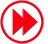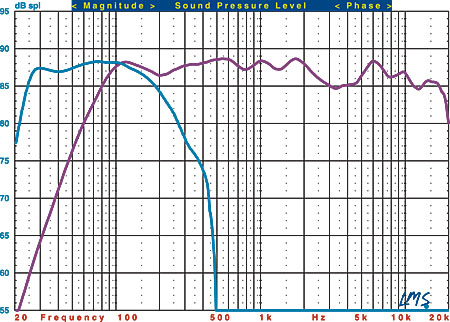# Surround Processor Reviews

## Anthem AVM 30 Pre/Pro, Anthem MCA 50 Amplifier, and KEF KHT 9000 ACE Speaker System HT Labs Measures

HT Labs Measures: Anthem AVM 30 Pre/ProAnalog frequency response in direct mode: -0.05 dB at 10 Hz; -0.01 dB at 20 Hz-0.09 dB at 20 kHz; -0.55 dB at 50 kHz

Analog frequency response with signal processing: -0.24 dB at 10 Hz; -0.06 dB at 20 Hz -0.03 dB at 20 kHz; -36.40 dB at 50 kHz

The above chart shows the frequency response of the left (aqua), center (green), LFE (purple), and left surround (red) channels at the preamp outputs of the Dolby Digital decoder. The left channel measures -0.03 decibels at 20 hertz and +0.09 dB at 20 kilohertz. The center channel measures -0.03 dB at 20 Hz and +0.08 dB at 20 kHz, and the left surround channel measures -0.03 dB at 20 Hz and +0.08 dB at 20 kHz. The LFE channel, normalized to the level at 40 Hz, is +0.07 dB at 20 Hz, reaches the upper -3dB point at 106 Hz, and reaches the upper -6dB point at 113 Hz.

Response from the multichannel input to the main output in direct mode measures -0.06 dB at 10 Hz, -0.01 dB at 20 Hz, -0.09 dB at 20 kHz, and -0.56 dB at 50 kHz. The analog THD+N is less than 0.021% at 1 kHz with a 100-millivolt input and the volume control set to -3.5. Crosstalk with a 100-mV input was -90.80 dB left to right and -91.93 dB right to left. The signal-to-noise ratio with a 100-mV input from 10 Hz to 24 kHz with "A" weighting was -122.14 dBrA.—MJP

HT Labs Measures: Anthem MCA 50 AmplifierAll channels driven into 8-ohm loads: 0.1% distortion at 193.3 watts 1% distortion at 222.8 watts

Frequency response: -0.04 dB at 10 Hz; -0.01 dB at 20 Hz +0.03 dB at 20 kHz; -0.94 dB at 50 kHz

This graph shows that the MCA 50's left amplifier channel, with two channels driving 8-ohm loads, reaches 0.1% distortion at 199.9 watts and 1% distortion at 232.3 watts. Into 4 ohms, the amplifier reaches 0.1% distortion at 344.5 watts and 1% distortion at 378.1 watts. An input level of 102.5 millivolts was required to produce an output of 2.83 volts into an 8-ohm load, indicating an overall gain of +28.86 decibels using the RCA input. When using the XLR input, a level of 103.0 millivolts was required to produce an output of 2.83 volts into an 8-ohm load, indicating an overall gain of +28.82 decibels.

THD+N from the amplifier was less than 0.002% at 1 kHz when driving 2.83 volts into an 8-ohm load using the RCA input. When using the XLR input under the same conditions, THD+N was less than 0.004%. Crosstalk at 1 kHz driving 2.83 volts into an 8-ohm load was -111.03 dB left to right and -107.75 dB right to left using the RCA inputs and -106.56 dB left to right and -108.09 dB right to left using the XLR inputs. The signal-to-noise ratio with 2.83 volts driving an 8-ohm load from 10 Hz to 24 kHz with "A" weighting was -114.74 dBrA using the RCA input and -107.85 using the XLR input.—MJP

HT Labs Measures: KEF KHT 9000 ACE Speaker SystemSatellite Sensitivity: 88 dB from 500 Hz to 2 kHz

This graph shows the quasi-anechoic (employing close-miking of all woofers) frequency response of the KHT 9000 satellite speakers (purple trace) and PSW 4000 subwoofer (blue trace). The satellites were measured at a distance of 1 meter with a 2.83-volt input.

The KHT 9000's listening-window response (a five-point average of axial and +/-15-degree horizontal and vertical responses) measures +0.65/-3.31 dB from 200 Hz to 10 kHz. When mounted horizontally for center-channel use, an average of axial and +/-15-degree horizontal responses measures +0.97/-3.16 dB from 200 Hz to 10 kHz. The -3dB point is at 80 Hz, and the -6dB point is at 67 Hz. Impedance reaches a minimum of 4.61 ohms at 121 Hz and a phase angle of -48.49 degrees at 3.2 kHz.

The PSW 4000's close-miked response, normalized to the level at 80 Hz, indicates that the lower -3dB point is at 24 Hz and the -6dB point is at 22 Hz. The upper -3dB point is at 181 Hz using the LFE input.—MJPARTICLE CONTENTSX